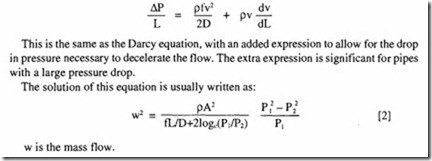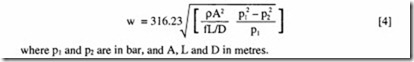# Energy and Efficiency:The complete isothermal formula

###### The complete isothermal formula

The equation for compressible flow, which takes into account that the pressure at the end of a long pipe may be significantly smaller than that at the beginning, is:This is correct for any consistent set of units. In particular, in conformity with the standard nomenclature, it applies where L, D and A are expressed in metres, P in N/m 2 and w in kg/s.

This solution is developed on the basis of the following assumptions:

• Isothermal flow

• No mechanical work done on the system

• The velocity is the average across the section

• The gas obeys perfect gas laws, ie the compressibility factor is unity

• Friction factor is constant

• The pipe is straight and level.

For gas flow it is permissible to add one further assumption:

• Forces due to acceleration of the flow can be neglected.

Equation  now becomes:

It can be seen that this is equivalent to the incompressible Darcy equation if the pressure difference between the ends is small. It can also be seen that if the pressure drop is less than 10% of the inlet pressure and is based on the conditions at either inlet or outlet, the formula is accurate to about 5 %, which is satisfactory for most purposes. If the pressure drop is more than 10% and less than 40%, the formula can still be used provided the average value of p is used. Note that this procedure may involve iteration if the first guess of pressure drop proves to be inaccurate. For long pipelines, where the pressure drop is greater than 40%, two techniques can be used; one is to divide the pipe into shorter sections and then by a process of iteration solve for the complete pipe; the other is to use equation (2]. The latter procedure cannot take into account differences in the value of p along the length. If a significant pressure drop of this magnitude is found to occur in a normal compressed air layout, there is quite obviously a fault in the design of the system which should be remedied.

Equation  expressed in the usual units becomes